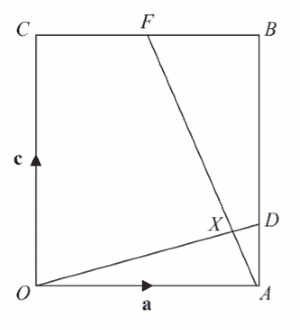# Λ and μ are scalars, find the value of λ and the value of μ

• MHB
• Help seeker
In summary, the figure shows a rectangle OABC with side lengths OA = a and OC = c. Point F is the midpoint of CB and point D is located on AB such that AD : DB = 2 : 3. Part (a) asks to find CF in terms of a and AD in terms of c, which can be determined using the given ratios. Part (b) involves finding the values of scalars λ and μ by setting up a coordinate system and using the equations of the lines OD and AF. Part (c) can be solved by using the intersection information from part (b). Finally, part (d) asks for the area of quadrilateral XDBF, which can be found byf

#### Help seekerFigure shows a rectangle OABC in which OA = a and OC = c. F is the midpoint of CB and D is the point on AB such that AD : DB = 2:3
(a) Find
_ _ _ _ _ __ (i) CF in terms of a
_ _ _ _ _ _ _ _ _ _ _ _ _ _ _ _ _ _ _ (ii) AD in terms of c

The lines OD and AF intersect at the point X Given that
OX = λOD and AX = μAF, where λ and μ are scalars,

(b) find the value of λ and the value of μ

Given that OX : XD = n:1

(c) find the value of n

Given also that I a l = 12 cm and l c I = 12.5 cm,

(d) find the area, in cm2, of quadrilateral XDBF .

D
part (a) is straightforward ... you should be able to use the given ratios to determine CF and AD in terms of a and c respectively.

part (b) ... let O be the origin, (0,0), of a Cartesian grid, A = (a,0), F = (a/2,c), etc.

OD has slope $\dfrac{|AD|}{a} \implies \text{ segment OD has equation } y = \dfrac{|AD|}{a} \cdot x$, where |AD| is in terms of c found in part (a)

AF has slope $-\dfrac{2c}{a} \implies \text{ segment AF has equation } y = -\dfrac{2c}{a} (x - a)$

using the two linear equations, determine the intersection, which will allow you to determine the values of $\lambda$ & $\mu$

part (c) should be easy to determine from the intersection information in working part (b)

part (d) ... area of XDBF = area of ABCO - CFAO - ADX
Don't leave it half-way
Plz complete it.

I would set up a coordinate system so that O is at the origin, A is at (a, 0), and C is at (0, c). Then B is at (a, c).
F is at (a/2, c) so the line from A to F is y= -(2c/a)(x- a)= -(2c/a)x+ 2c. D is the point on AB such that AD : DB = 2:3 so if we take D to be (a, x), AD= c- x and DB= x. That is, AD/DB=x/(c- x)= 2/3. 3x=2(c- x)= 2c- 2x. 5x= 2c so x= (2/5)c. D is at (a, (2/5)c). The line from O to D is y= ((2/5)c/a)x. X is the intersection of the two lines so y= -(2c/a)x+ 2c= ((2/5)c/a)x. 2c= ((2/5+ 2)(c/a)x= (12/5)(c/a)x. x= (5/12)(a/c)(2c)= (5/6)a.
y= (2/5)(c/a)(5/6)a= c/3.

X is the point ((5/6)a, c/3).https://mathhelpboards.com/account/

OX= sqrt((25/36)a^2+ c^2/9)= sqrt{25a^2/36+ 4c^2/36}= sqrt(25a^2+ 4c^2)/6.
OD= sqrt(a^2+ 4c^2/25)= sqrt(25a^2+4c^2)/5.

AX= sqrt(a^2/36+ c^2/9)= sqrt((a^2/36+ 4c^2/36)= sqrt(a^2+ 4c^2)/6.
AF= sqrt(a^2/4+ c^2)= sqrt(a^2+ 4a)/2.

I would set up a coordinate system so that O is at the origin, A is at (a, 0), and C is at (0, c). Then B is at (a, c).
F is at (a/2, c) so the line from A to F is y= -(2c/a)(x- a)= -(2c/a)x+ 2c. D is the point on AB such that AD : DB = 2:3 so if we take D to be (a, x), AD= c- x and DB= x. That is, AD/DB=x/(c- x)= 2/3. 3x=2(c- x)= 2c- 2x. 5x= 2c so x= (2/5)c. D is at (a, (2/5)c). The line from O to D is y= ((2/5)c/a)x. X is the intersection of the two lines so y= -(2c/a)x+ 2c= ((2/5)c/a)x. 2c= ((2/5+ 2)(c/a)x= (12/5)(c/a)x. x= (5/12)(a/c)(2c)= (5/6)a.
y= (2/5)(c/a)(5/6)a= c/3.

X is the point ((5/6)a, c/3).https://mathhelpboards.com/account/

OX= sqrt((25/36)a^2+ c^2/9)= sqrt{25a^2/36+ 4c^2/36}= sqrt(25a^2+ 4c^2)/6.
OD= sqrt(a^2+ 4c^2/25)= sqrt(25a^2+4c^2)/5.

AX= sqrt(a^2/36+ c^2/9)= sqrt((a^2/36+ 4c^2/36)= sqrt(a^2+ 4c^2)/6.
AF= sqrt(a^2/4+ c^2)= sqrt(a^2+ 4a)/2.
Tnx

Tnx
Solved